## Teach your students how to add, subtract multiply and divide fractions with these engaging lessons, activities, and assessments.

Explore strategies and resources for the following:

When teaching students operations with fractions it is important to:

✅give your students many opportunities to practice (math stations, homework, classwork, early finisher activities)

✅expose your students to different activities (fun mazes riddles and coloring pages)

✅ have your students apply their understanding by solving real-world word problems (task cards and graphic organizers)

✅ assess your student's understanding (printed or digital assessments)

# Here are a few activities that I include in my Fractions Math Unit.

## Add and Subtract Fractions ActivitiesGraphic Organizers, Error Analysis, Maze, Riddle, Coloring Activity

The Add and Subtract Fractions Unit Resource BUNDLE includes 10 error analysis activities, 10 problem solving graphic organizers, 1 maze, 1 riddle, 1 coloring activity (over 45 skills practice and real-world word problems).

This unit resource bundle includes:

Each page has real-world word problems that is solved incorrectly. Students have to identify the error, provide the correct solution and share a helpful strategy for solving the problem.Add and Subtract Fractions Error Analysis Activity

10 Problem Solving Graphic Organizers - Each worksheet presents students with a real-world problem. Students must then organize the information using a problem-solving graphic organizer. Students are prompted to identify the important information in the word problem, solve, justify their work and explain their solution.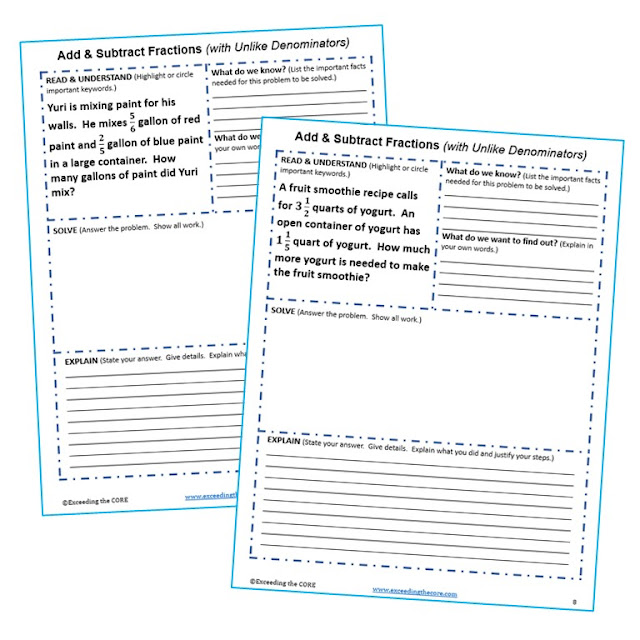Add and Subtract Fractions Problem-Solving Graphic Organizer

A maze, riddle and coloring page is also included. All 3 of these activities allow students to practice this skill while incorporating fun into the classroom!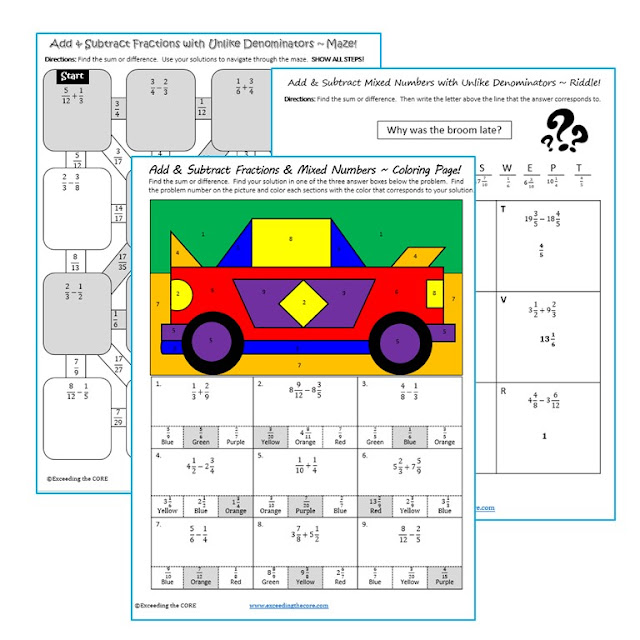Add and Subtract Fractions Maze Riddle and Coloring Page

Topics included:

✔ Add and subtract fractions with unlike denominators.

✔ Add and subtract mixed numbers with unlike denominators.

✔ Rename to find the difference between two mixed numbers.

## MULTIPLY & DIVIDE FRACTIONS BUNDLE

Task Cards, Error Analysis, Word Problems with Graphic Organizers, Maze, Riddle, Coloring Page, Guided Notes, Homework Practice

This Multiply and Divide Fractions UNIT RESOURCE BUNDLE includes 40 task cards, 10 error analysis activities and 10 problem solving graphic organizers, 1 maze, 1 riddle, 1 coloring page activity, 3 guided notes pages, 5 homework practice pages (over 150 skills practice and real-world word problems).

This unit resource bundle includes:

Each page has a real-world word problem that is solved incorrectly. Students have to identify the error, provide the correct solution and share a helpful strategy for solving the problem.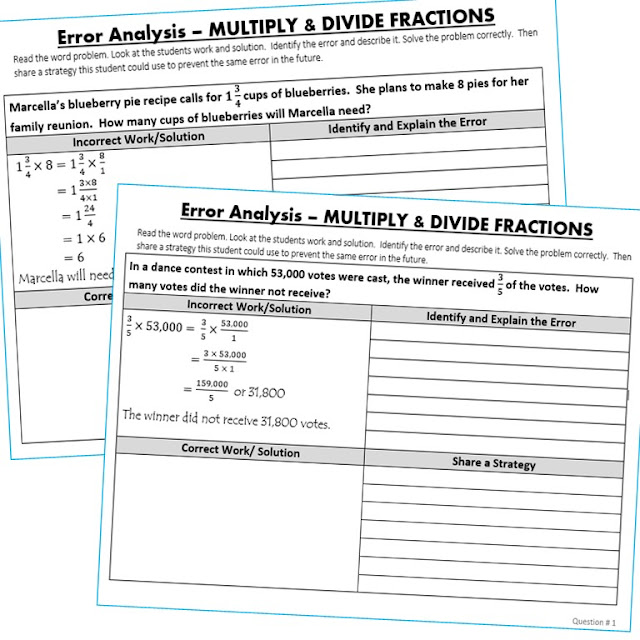Multiply and Divide Fractions Find the Error Activity

Each task card has a real-world word problem. Students can work on these cards individually, with partners or in groups.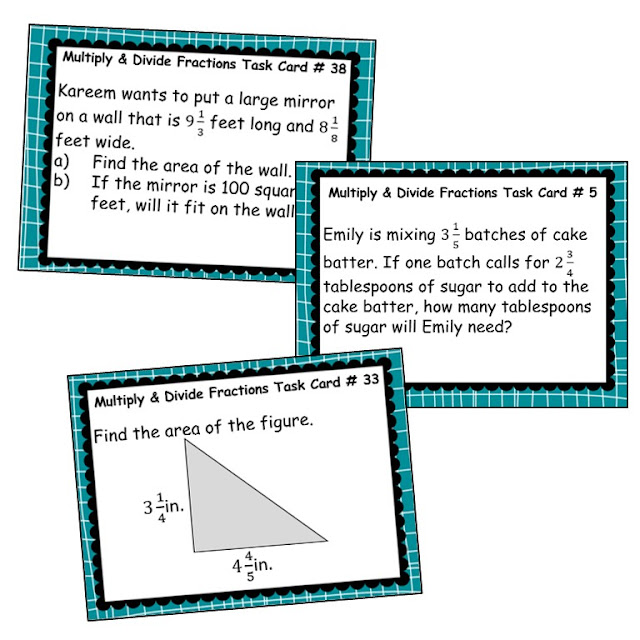Multiply and Divide Fractions Word Problems Task Cards

Each worksheet presents students with a real-world word problem. Students must then organize the information using a problem-solving graphic organizer. Students are prompted to identify the important information in the word problem, solve, justify their work and explain their solution.Multiply and Divide Fractions Word Problem Graphic Organizer

A maze, riddle and coloring page is also included. All 3 of these activities allow students to practice this skill while incorporating fun into the classroom!Multiply and Divide Fractions Maze, Riddle, Coloring Page

These guided notes include essential vocabulary, key concepts and practice questions. Students answer the questions, and then color, doodle or embellish these notes. These guided notes are perfect for interactive notebooks or mini-anchor charts.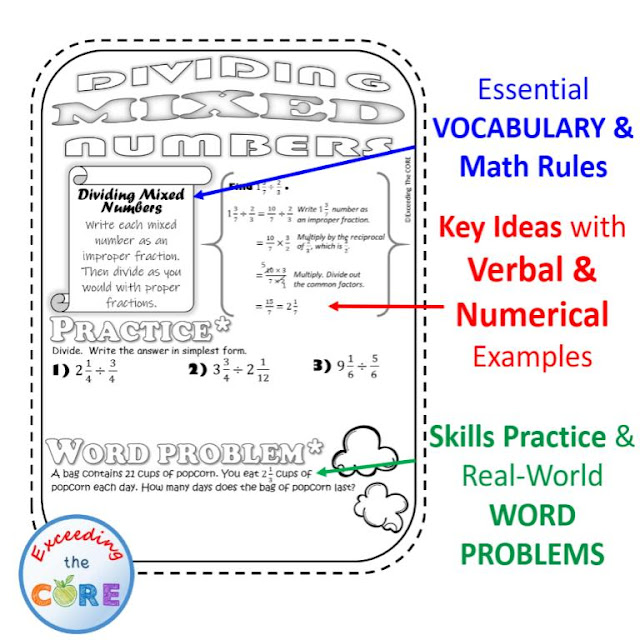Multiply and Divide Fractions Guided Notes

Each worksheet includes two sections, Skills Practice and Problems Solving. The SKILLS PRACTICE section of each worksheet includes 6 computational problems to help students sharpen their skills. The WORD PROBLEM section of each worksheet allows students to apply their understanding of the topic to solve 4 real-world problems.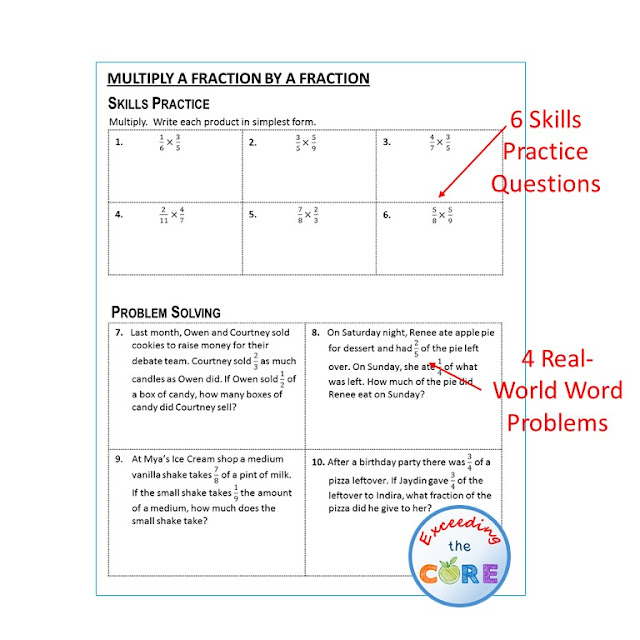Multiply and Divide Fractions Homework Practice Worksheets

## Operations with Fractions Digital Assessments (Google Quizzes)

Use the digital resources in this Operations with Fractions Digital Assessment Bundle to assess your students' understanding of Adding, Subtracting, Multiplying, and Dividing Fractions.

What is Included:

This BUNDLE includes three quizzes. Each quiz includes 10 questions. They are self-grading quizzes and were created with Google Forms. This is perfect for GOOGLE Classroom, remote learning, or distance learning.

Topics Covered:

Benefits of this Resource:

Things to Know:

• This resource was created using Google Forms.
• You and your students must have a free Google account in order to access this activity.
• You can find many more GOOGLE APPS like this to support your remote/online instruction.

Ways to Use this Resource:

• This resource is perfect for homework, computer centers, online testing practice, pre-assessments, post-assessments, RTI (Response to Intervention), and remote / distance learning.